# Index Section A Section B Section C Section

• Slides: 39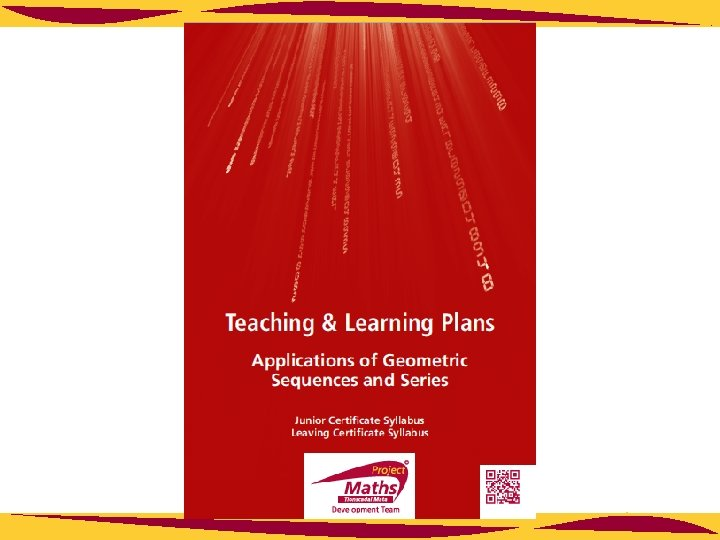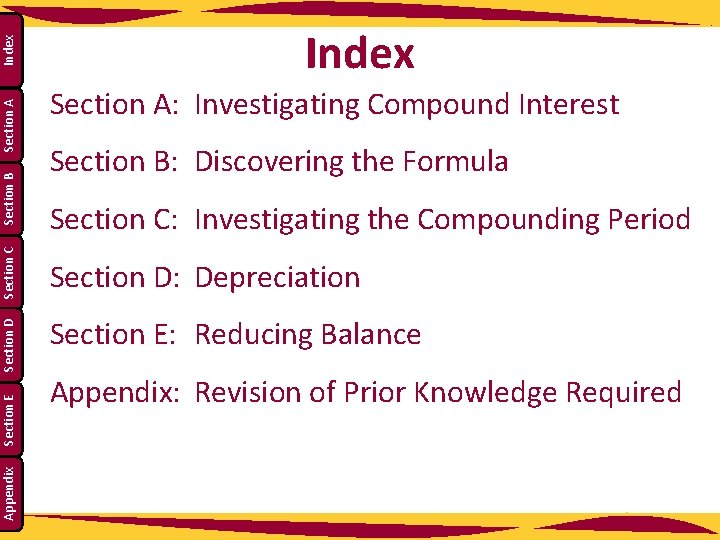Index Section A Section B Section C Section D Section E Appendix Index Section A: Investigating Compound Interest Section B: Discovering the Formula Section C: Investigating the Compounding Period Section D: Depreciation Section E: Reducing Balance Appendix: Revision of Prior Knowledge Required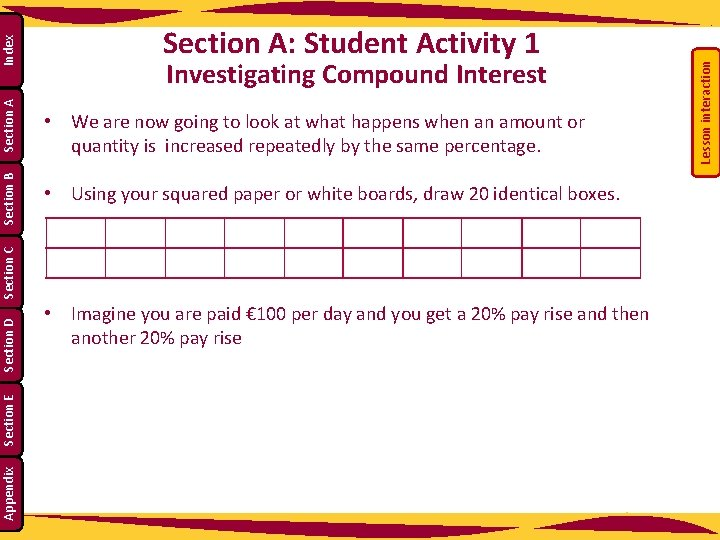• We are now going to look at what happens when an amount or quantity is increased repeatedly by the same percentage. • Using your squared paper or white boards, draw 20 identical boxes. Section D Section E Appendix • Imagine you are paid € 100 per day and you get a 20% pay rise and then another 20% pay rise Lesson interaction Index Section A Investigating Compound Interest Section C Section B Section A: Student Activity 1Investigating Compound Interest 4. What is the value of the second shaded area? _______________ Appendix Lesson interaction 2. Then, using another colour, add 20% to the original shaded area. Section E Section C 1. If each block represents € 10, shade in € 100. Section D Section B Section A Index Section A: Student Activity 1 3. Finally, using a third colour, add 20% of the entire shaded area. 5. What is the value of the third shaded area? ________________ 6. Why do they not have the same amount? ________________ 4 2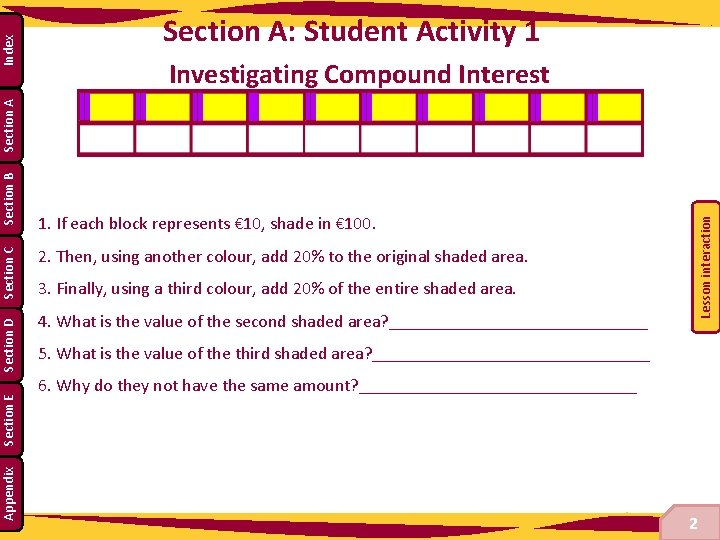Investigating Compound Interest Appendix Section E 1. If each block represents € 10, shade in € 100. Lesson interaction Section D Section C Section B Section A Index Section A: Student Activity 1 2. Then, using another colour, add 20% to the original shaded area. 3. Finally, using a third colour, add 20% of the entire shaded area. 4. What is the value of the second shaded area? _______________ 5. What is the value of the third shaded area? ________________ 6. Why do they not have the same amount? ________________ 5 2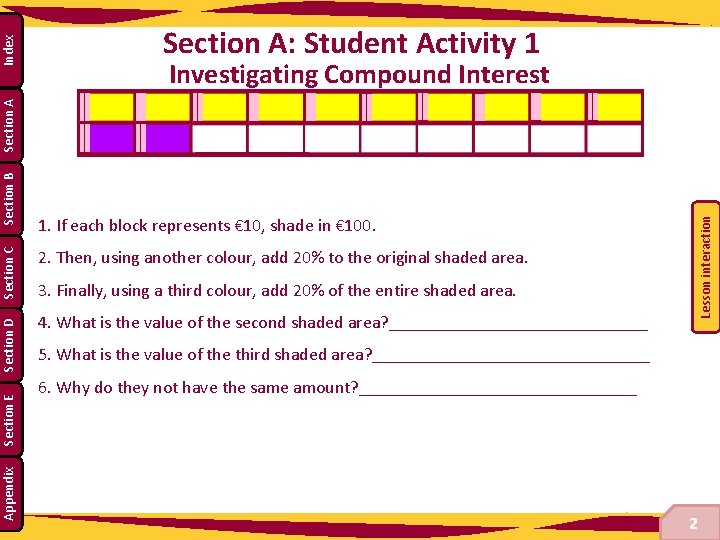Investigating Compound Interest Appendix Section E 1. If each block represents € 10, shade in € 100. Lesson interaction Section D Section C Section B Section A Index Section A: Student Activity 1 2. Then, using another colour, add 20% to the original shaded area. 3. Finally, using a third colour, add 20% of the entire shaded area. 4. What is the value of the second shaded area? _______________ 5. What is the value of the third shaded area? ________________ 6. Why do they not have the same amount? ________________ 6 2Lesson interaction 8. Can you find a way of getting the value for day 10 without having to do the table to day 10? ___________________________________ __________________ 9. Use the diagram on the right to graph time against amount. Is the relationship linear? Lesson interaction Index Appendix Section E Section D Section C Section B Section A 7. Complete the following table and investigate the patterns which appear.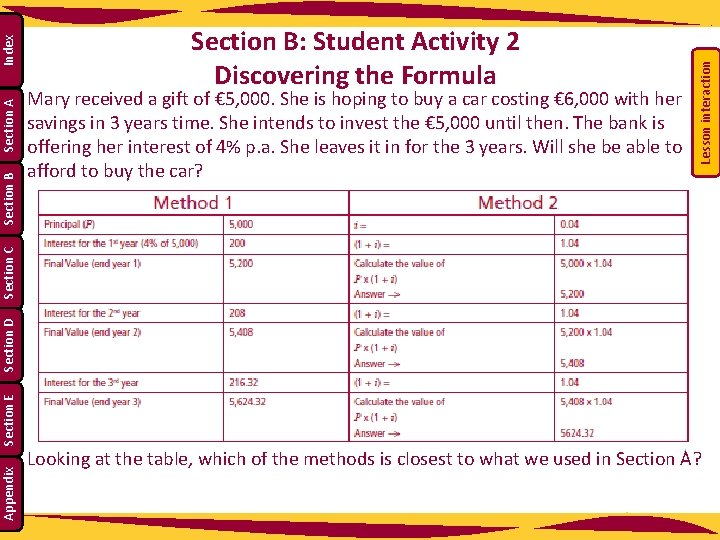Lesson interaction Index Section A Mary received a gift of € 5, 000. She is hoping to buy a car costing € 6, 000 with her savings in 3 years time. She intends to invest the € 5, 000 until then. The bank is offering her interest of 4% p. a. She leaves it in for the 3 years. Will she be able to afford to buy the car? Appendix Section E Section D Section C Section B: Student Activity 2 Discovering the Formula Looking at the table, which of the methods is closest to what we used in Section A?• You may use the following table. Time in years Amount Increase by 0 5, 000 4% 1 4% 2 4% 3 4% Total decimal Pattern/ Total amount of money received per year • Write out the entire sum with the final answer. • Now write it out in words, explaining what each term means. • Each explanation is correct but it might be easier if we all used the same terminology and abbreviations. Let’s look at page 30 of the Formulae and Tables book. Lesson interaction Index Section A Section B Section C Section D Section E Appendix • Work in groups and using the same idea as in Section A, try and get the general rule or formula for this instance.Using € 5, 000 x (1. 04)3 = € 5, 624. 32 and the formula in the tables, rewrite the equation to look like the original formula. Lesson interaction Index Section A Section B Section C Section D Section E Appendix Now write out the general rule for Section A in the same order as the formula in the Formulae and Tables book.Section E Appendix 4. Fill in the appropriate values: a. Principal = ________________ b. Total interest = ______________ c. Final value = _______________ 5. Using the formula from the tables, write out the entire equation in the same order. __________________________________________________________________ Lesson interaction Index Section A Section B 3. Write the value of the interest for the 1 st, 2 nd and 3 rd years in the boxes below. Section D 2. Write a sentence explaining whether or not Mary can afford the car from her bank savings. ____________________________________________________________ Section C 1. Does this amount to the proof of the formula? Discuss. __________________________________________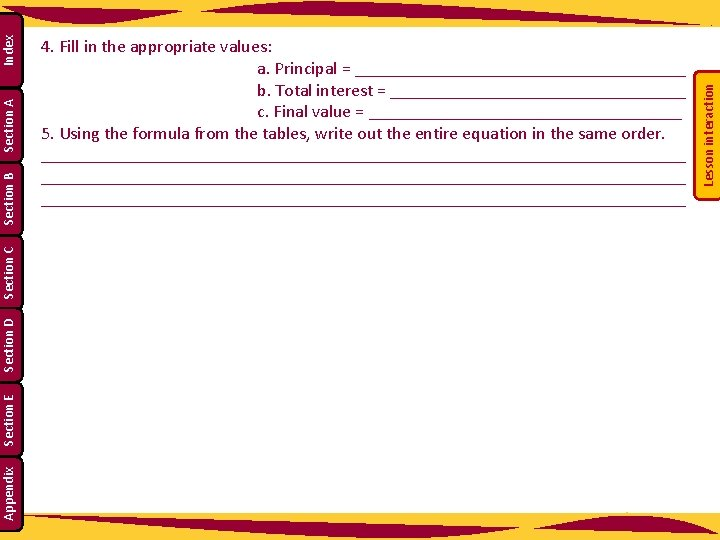Lesson interaction Index Section A Section B Section C Section D Section E Appendix 4. Fill in the appropriate values: a. Principal = ___________________ b. Total interest = _________________ c. Final value = __________________ 5. Using the formula from the tables, write out the entire equation in the same order. ________________________________________________________________________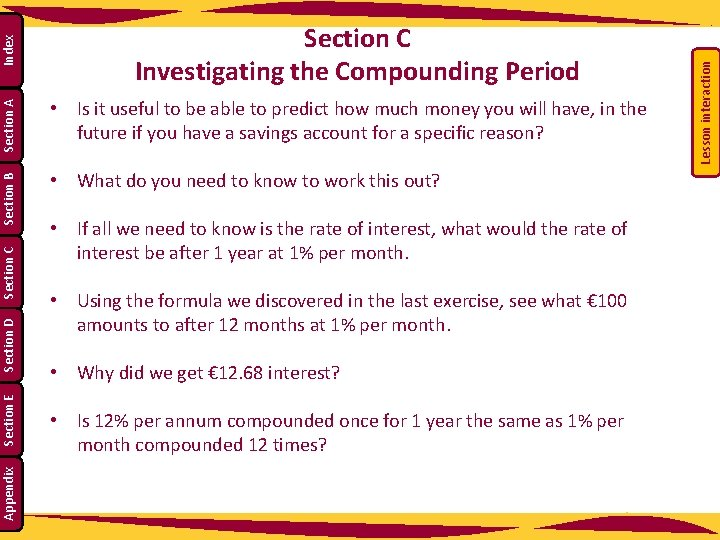Section D Section E Appendix • If all we need to know is the rate of interest, what would the rate of interest be after 1 year at 1% per month. • Using the formula we discovered in the last exercise, see what € 100 amounts to after 12 months at 1% per month. • Why did we get € 12. 68 interest? • Is 12% per annum compounded once for 1 year the same as 1% per month compounded 12 times? Lesson interaction Index Section A • What do you need to know to work this out? Section C • Is it useful to be able to predict how much money you will have, in the future if you have a savings account for a specific reason? Section B Section C Investigating the Compounding Period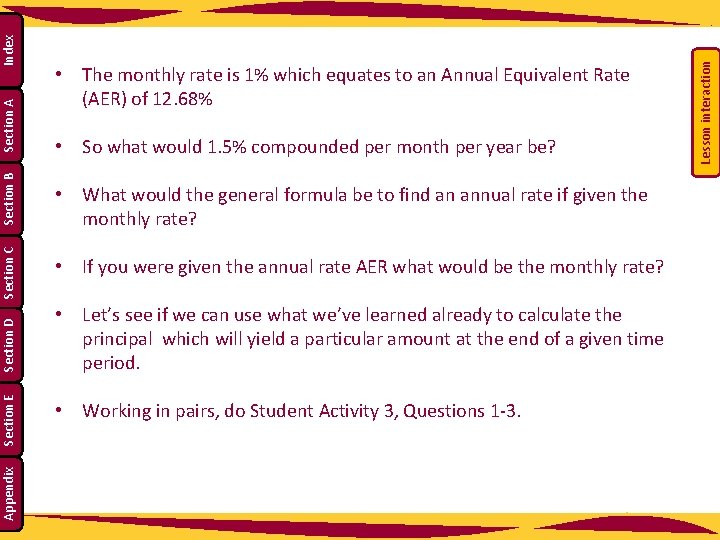• If you were given the annual rate AER what would be the monthly rate? • Let’s see if we can use what we’ve learned already to calculate the principal which will yield a particular amount at the end of a given time period. Appendix Section E Section C • What would the general formula be to find an annual rate if given the monthly rate? • Working in pairs, do Student Activity 3, Questions 1 -3. Lesson interaction Index Section A • So what would 1. 5% compounded per month per year be? Section D Section B • The monthly rate is 1% which equates to an Annual Equivalent Rate (AER) of 12. 68%1. Fill as many variables as you can into the table below Section D Section E Appendix 2. Use the boxes below to find the value of P Lesson interaction Index Section A John wants to have € 10, 000 saved in 10 years time to pay for his child’s education. The bank is offering him an annual interest rate of 7%. How much money would he need to put in now in order to have € 10, 000 in 10 years time? Round off to the nearest € 100. Section C Section B Section C: Student Activity 3 Investigating the Compounding Period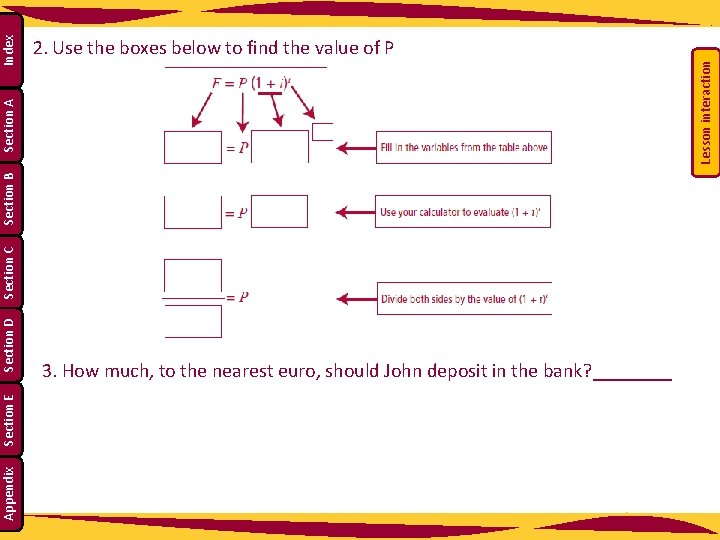Appendix Section E Section D Lesson interaction Section C Section B Section A Index 2. Use the boxes below to find the value of P 3. How much, to the nearest euro, should John deposit in the bank? ____Section D Section E Appendix Complete the following: Lesson interaction Index Section A Using the relevant nth root, find the rate of interest applied to get the final sum from the principal sum. Remember that you are working with money so rounding to the nearest cent has occurred. The rates should be written to one decimal place (AER) for this exercise. Example: Section C Section B 4. The formula for compound interest is F = P (1 + i)t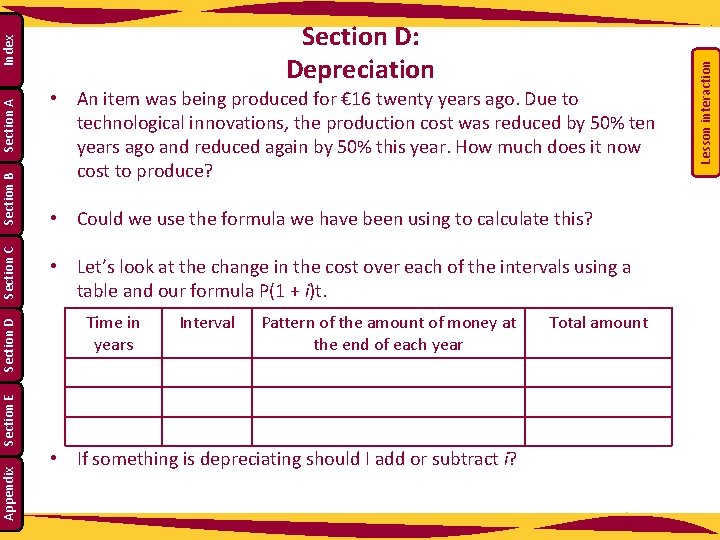• Could we use the formula we have been using to calculate this? • Let’s look at the change in the cost over each of the intervals using a table and our formula P(1 + i)t. Time in years Interval Pattern of the amount of money at the end of each year Appendix • If something is depreciating should I add or subtract i? Total amount Lesson interaction • An item was being produced for € 16 twenty years ago. Due to technological innovations, the production cost was reduced by 50% ten years ago and reduced again by 50% this year. How much does it now cost to produce? Section E Section D Section C Section B Section A Index Section D: Depreciation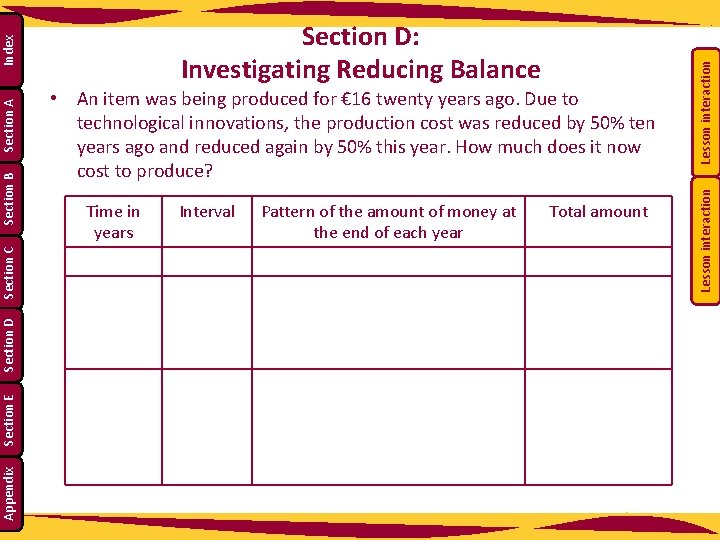Appendix Section E Section D Time in years Interval Pattern of the amount of money at the end of each year Total amount Lesson interaction • An item was being produced for € 16 twenty years ago. Due to technological innovations, the production cost was reduced by 50% ten years ago and reduced again by 50% this year. How much does it now cost to produce? Lesson interaction Section C Section B Section A Index Section D: Investigating Reducing Balance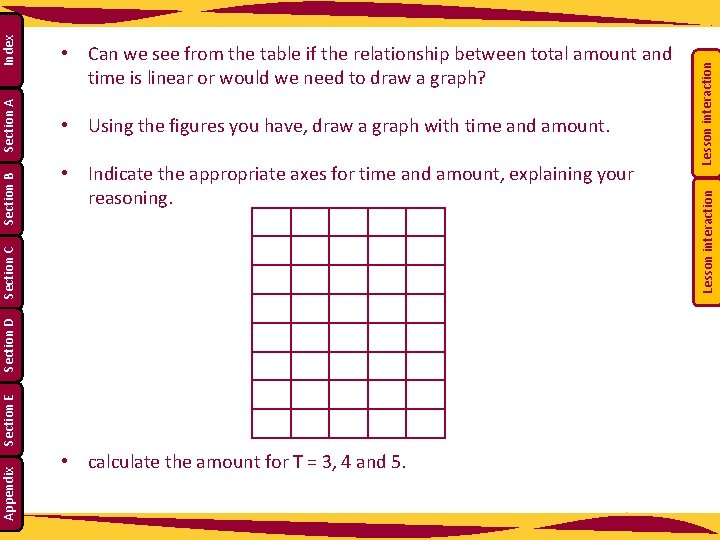Section D Section E Appendix • calculate the amount for T = 3, 4 and 5. Lesson interaction • Indicate the appropriate axes for time and amount, explaining your reasoning. Lesson interaction Index Section A • Using the figures you have, draw a graph with time and amount. Section C Section B • Can we see from the table if the relationship between total amount and time is linear or would we need to draw a graph?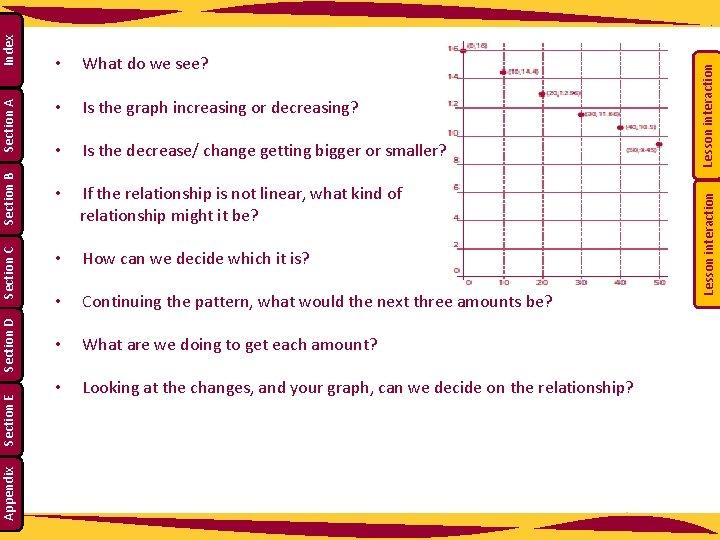• Is the graph increasing or decreasing? • Is the decrease/ change getting bigger or smaller? • If the relationship is not linear, what kind of relationship might it be? • How can we decide which it is? • Continuing the pattern, what would the next three amounts be? • What are we doing to get each amount? • Looking at the changes, and your graph, can we decide on the relationship? Lesson interaction What do we see? Lesson interaction Index Section A Section B Section C Section D Section E Appendix •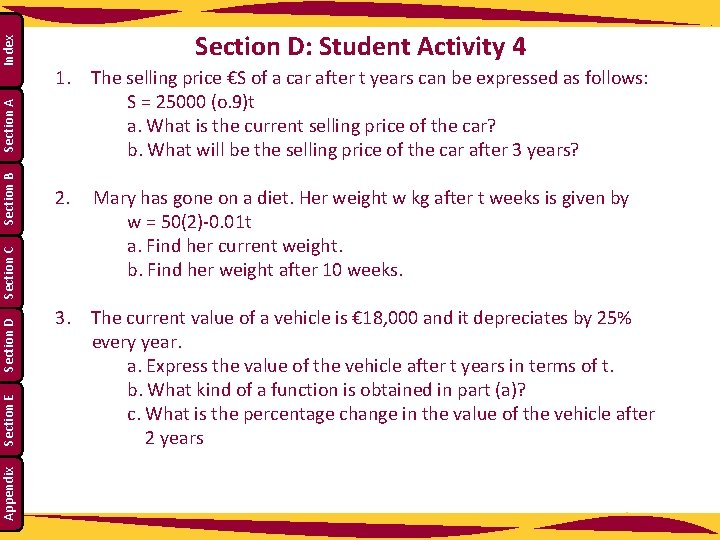Index Section A Section B Section C Section D Section E Appendix Section D: Student Activity 4 1. The selling price €S of a car after t years can be expressed as follows: S = 25000 (o. 9)t a. What is the current selling price of the car? b. What will be the selling price of the car after 3 years? 2. Mary has gone on a diet. Her weight w kg after t weeks is given by w = 50(2)-0. 01 t a. Find her current weight. b. Find her weight after 10 weeks. 3. The current value of a vehicle is € 18, 000 and it depreciates by 25% every year. a. Express the value of the vehicle after t years in terms of t. b. What kind of a function is obtained in part (a)? c. What is the percentage change in the value of the vehicle after 2 yearsIndex Section A Section B Section C Section D Section E Appendix 4. Conor makes a New Year’s resolution and plans to keep fit for the coming year. His target is to decrease his current weight of 80 kg by 1% each week in the coming months. If Conor reaches his target each week, a. Express his weight after t weeks in terms of t. b. Find his weight after 4 weeks. c. Find the percentage change in his weight after 4 weeksDavid and Michael are going on the school tour this year. They are each taking out a loan of € 600, which they hope to pay off over the next year. Their bank is charging a monthly interest rate of 1. 5% on loans. David says that with his part time work at present he will be able to pay € 100 for the first 4 months but will only be able to pay off € 60 a month after that. Michael says that he can only afford to pay € 60 for the first 4 months and then € 100 after that. Michael reckons that they are both paying the same amount for the loan. Why? ____________________________________________________________ Note: This problem is posed based on the following criteria: (a) A loan is taken out (b) After 1 month interest is added on (c) The person then makes his/her monthly repayment. This process is then repeated until the loan is fully paid off. Lesson interaction Index Section A Section B Section C Section D Section E Appendix Section E: Student Activity 5 Reducing Balance0 Monthly Total € 600. 00 1 € 509. 00 € 7. 64 € 100. 00 1 € 549. 00 € 8. 24 € 60. 00 2 € 416. 64 € 6. 25 € 100. 00 2 € 497. 24 € 7. 46 € 60. 00 3 € 322. 88 € 4. 84 € 100. 00 3 € 444. 69 € 6. 67 € 60. 00 4 € 227. 73 € 3. 42 € 60. 00 4 € 391. 36 € 5. 87 € 100. 00 5 € 171. 14 € 2. 57 € 60. 00 5 € 297. 23 € 4. 46 € 100. 00 6 € 113. 71 € 1. 71 € 60. 00 6 € 201. 72 € 3. 03 € 100. 00 7 € 55. 42 € 0. 83 € 56. 25 7 € 104. 72 € 1. 57 € 100. 00 8 8 € 6. 29 € 0. 09 € 6. 38 9 9 10 10 Time Interest Payments Time € 9. 00 € 100. 00 0 Monthly Total € 600. 00 Michael Interest Payments € 9. 00 € 60. 00 1. What do David and Michael have in common at the beginning of the loan period? ____________________________ Lesson interaction Index Section A Section B Section C Section D Section E Appendix David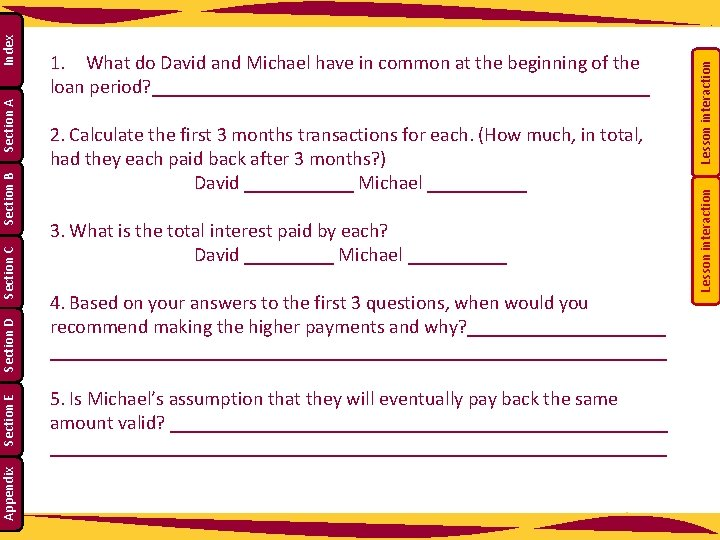3. What is the total interest paid by each? David _____ Michael _____ 4. Based on your answers to the first 3 questions, when would you recommend making the higher payments and why? _________________________________________ 5. Is Michael’s assumption that they will eventually pay back the same amount valid? ________________________________________________________ Lesson interaction 2. Calculate the first 3 months transactions for each. (How much, in total, had they each paid back after 3 months? ) David ______ Michael _____ Lesson interaction Index Section A Section B Section C Section D Section E Appendix 1. What do David and Michael have in common at the beginning of the loan period? _________________________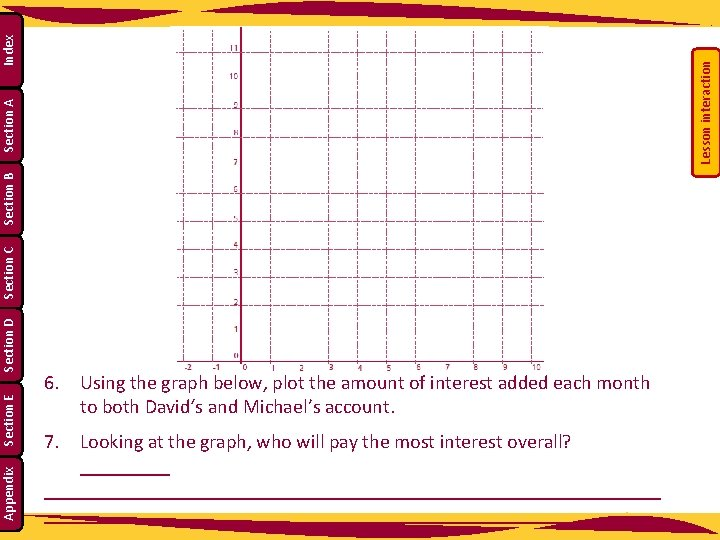Lesson interaction Index Section A Section B Section C Section D Section E Appendix 6. Using the graph below, plot the amount of interest added each month to both David‘s and Michael’s account. 7. Looking at the graph, who will pay the most interest overall? ____________________________________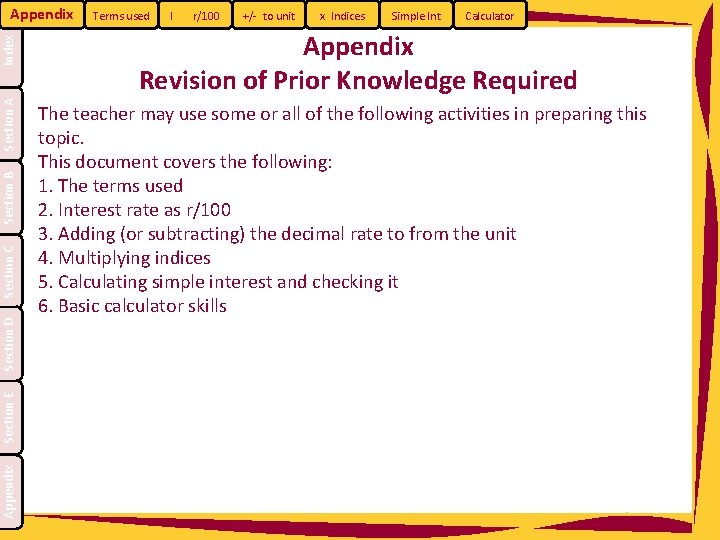Appendix Section E Section D Section C Section B Section A Index Appendix Terms used I r/100 +/- to unit x Indices Simple Int Calculator Appendix Revision of Prior Knowledge Required The teacher may use some or all of the following activities in preparing this topic. This document covers the following: 1. The terms used 2. Interest rate as r/100 3. Adding (or subtracting) the decimal rate to from the unit 4. Multiplying indices 5. Calculating simple interest and checking it 6. Basic calculator skills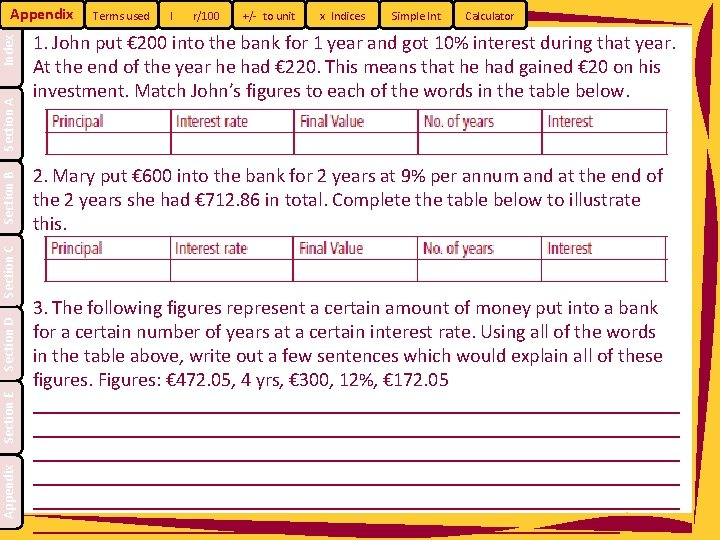Appendix Section E Section D Section C Section B Section A Index Appendix Terms used I r/100 +/- to unit x Indices Simple Int Calculator 1. John put € 200 into the bank for 1 year and got 10% interest during that year. At the end of the year he had € 220. This means that he had gained € 20 on his investment. Match John’s figures to each of the words in the table below. 2. Mary put € 600 into the bank for 2 years at 9% per annum and at the end of the 2 years she had € 712. 86 in total. Complete the table below to illustrate this. 3. The following figures represent a certain amount of money put into a bank for a certain number of years at a certain interest rate. Using all of the words in the table above, write out a few sentences which would explain all of these figures. Figures: € 472. 05, 4 yrs, € 300, 12%, € 172. 05 _________________________________________________________________ _________________________________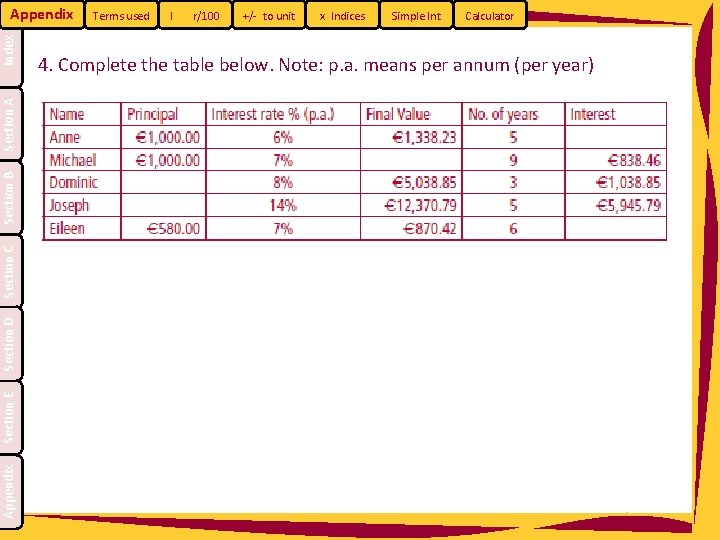Appendix Section E Section D Section C Section B Section A Index Appendix Terms used I r/100 +/- to unit x Indices Simple Int Calculator 4. Complete the table below. Note: p. a. means per annum (per year)Appendix Section E Section D Section C Section B Section A Index Appendix Terms used I r/100 +/- to unit x Indices Simple Int Calculator Interest rate as r/100 A percentage is a fraction having a denominator of 100. Therefore 9% means 9/100 which is 0. 09 as a decimal. Therefore an annual interest rate of 9% could be written as a decimal as 0. 09.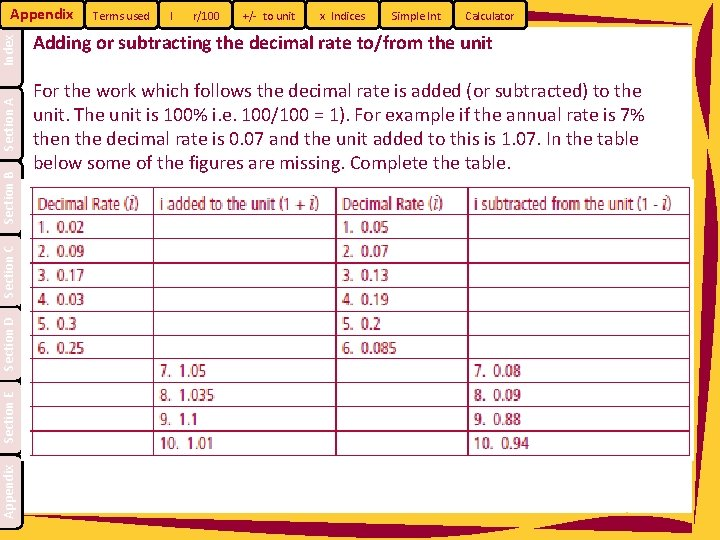Appendix Section E Section D Section C Section B Section A Index Appendix Terms used I r/100 +/- to unit x Indices Simple Int Calculator Adding or subtracting the decimal rate to/from the unit For the work which follows the decimal rate is added (or subtracted) to the unit. The unit is 100% i. e. 100/100 = 1). For example if the annual rate is 7% then the decimal rate is 0. 07 and the unit added to this is 1. 07. In the table below some of the figures are missing. Complete the table.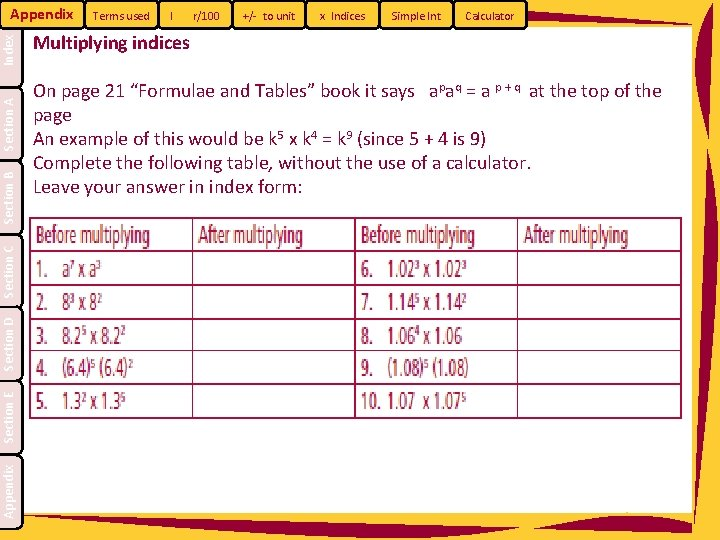Appendix Section E Section D Section C Section B Section A Index Appendix Terms used I r/100 +/- to unit x Indices Simple Int Calculator Multiplying indices On page 21 “Formulae and Tables” book it says apaq = a p + q at the top of the page An example of this would be k 5 x k 4 = k 9 (since 5 + 4 is 9) Complete the following table, without the use of a calculator. Leave your answer in index form: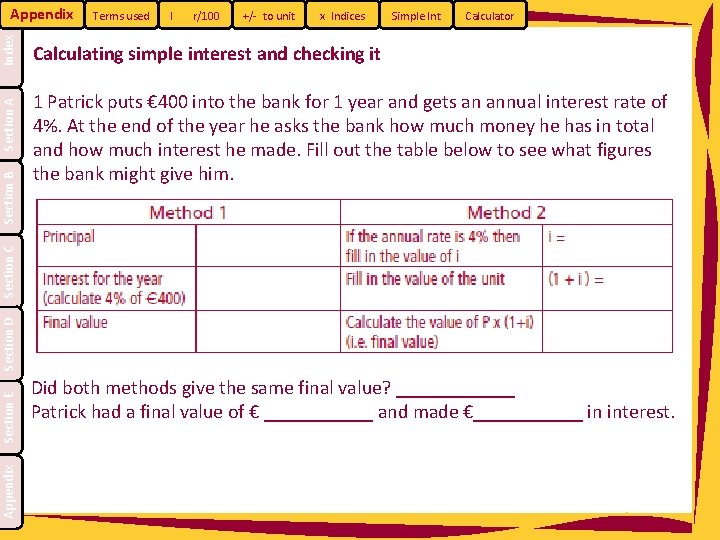Terms used I r/100 +/- to unit x Indices Simple Int Calculator Calculating simple interest and checking it 1 Patrick puts € 400 into the bank for 1 year and gets an annual interest rate of 4%. At the end of the year he asks the bank how much money he has in total and how much interest he made. Fill out the table below to see what figures the bank might give him. Appendix Section E Section D Section C Section B Section A Index Appendix Did both methods give the same final value? ______ Patrick had a final value of € ______ and made €______ in interest.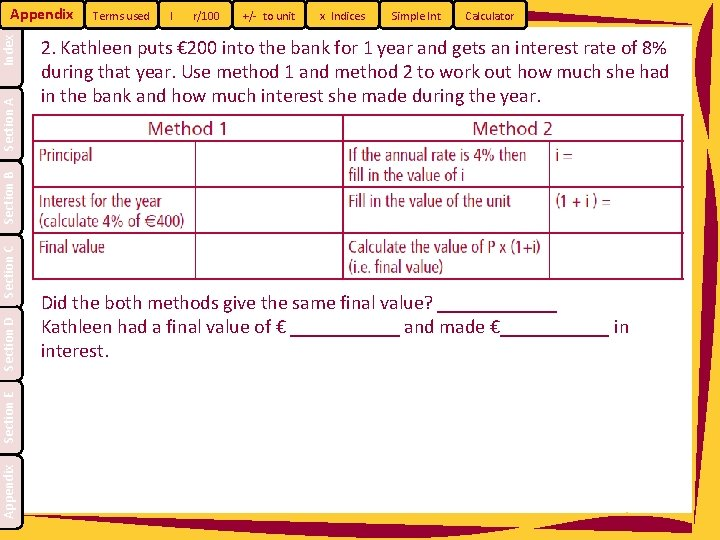Terms used I r/100 +/- to unit x Indices Simple Int Calculator 2. Kathleen puts € 200 into the bank for 1 year and gets an interest rate of 8% during that year. Use method 1 and method 2 to work out how much she had in the bank and how much interest she made during the year. Appendix Section E Section D Section C Section B Section A Index Appendix Did the both methods give the same final value? ______ Kathleen had a final value of € ______ and made €______ in interest.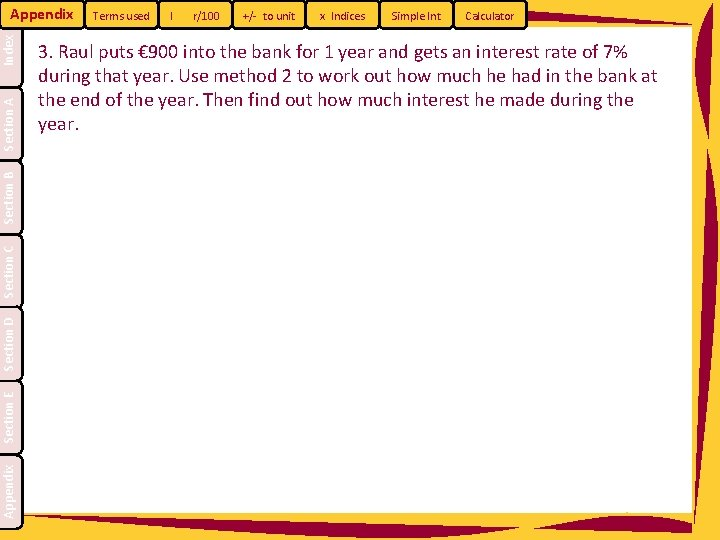Appendix Section E Section D Section C Section B Section A Index Appendix Terms used I r/100 +/- to unit x Indices Simple Int Calculator 3. Raul puts € 900 into the bank for 1 year and gets an interest rate of 7% during that year. Use method 2 to work out how much he had in the bank at the end of the year. Then find out how much interest he made during the year.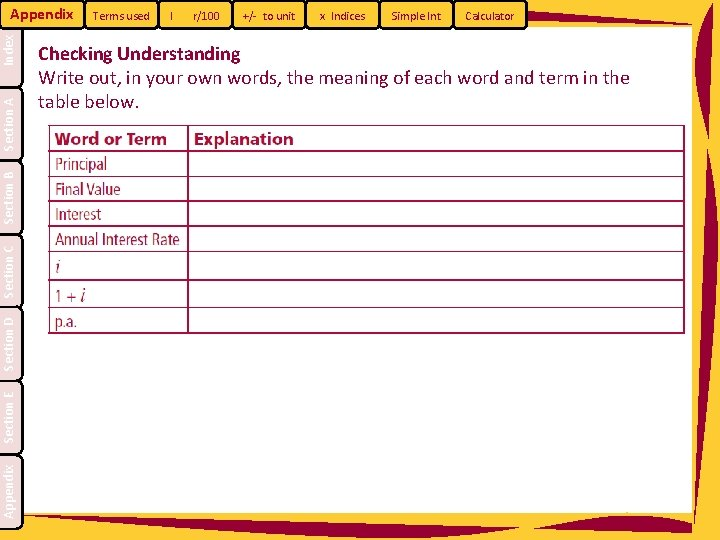Appendix Section E Section D Section C Section B Section A Index Appendix Terms used I r/100 +/- to unit x Indices Simple Int Calculator Checking Understanding Write out, in your own words, the meaning of each word and term in the table below.Appendix Section E Section D Section C Section B Section A Index Appendix Terms used I r/100 +/- to unit x Indices Simple Int Basic Calculator Skills Evaluate the following using your calculator: Calculator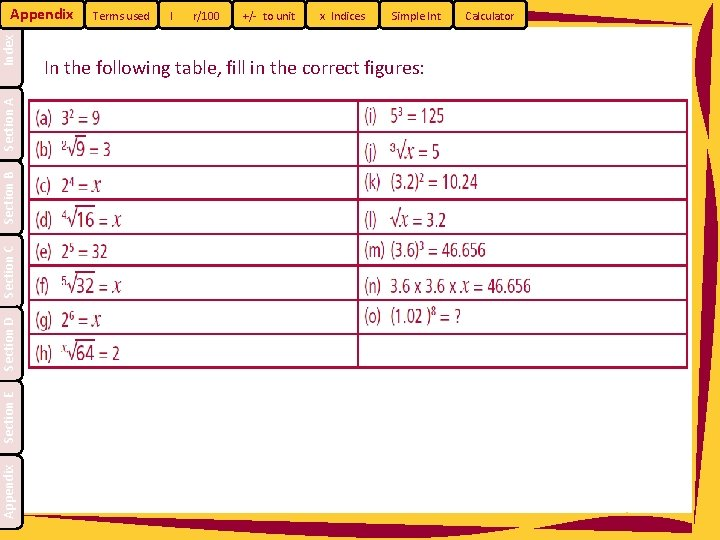Appendix Section E Section D Section C Section B Section A Index Appendix Terms used I r/100 +/- to unit x Indices Simple Int In the following table, fill in the correct figures: Calculator